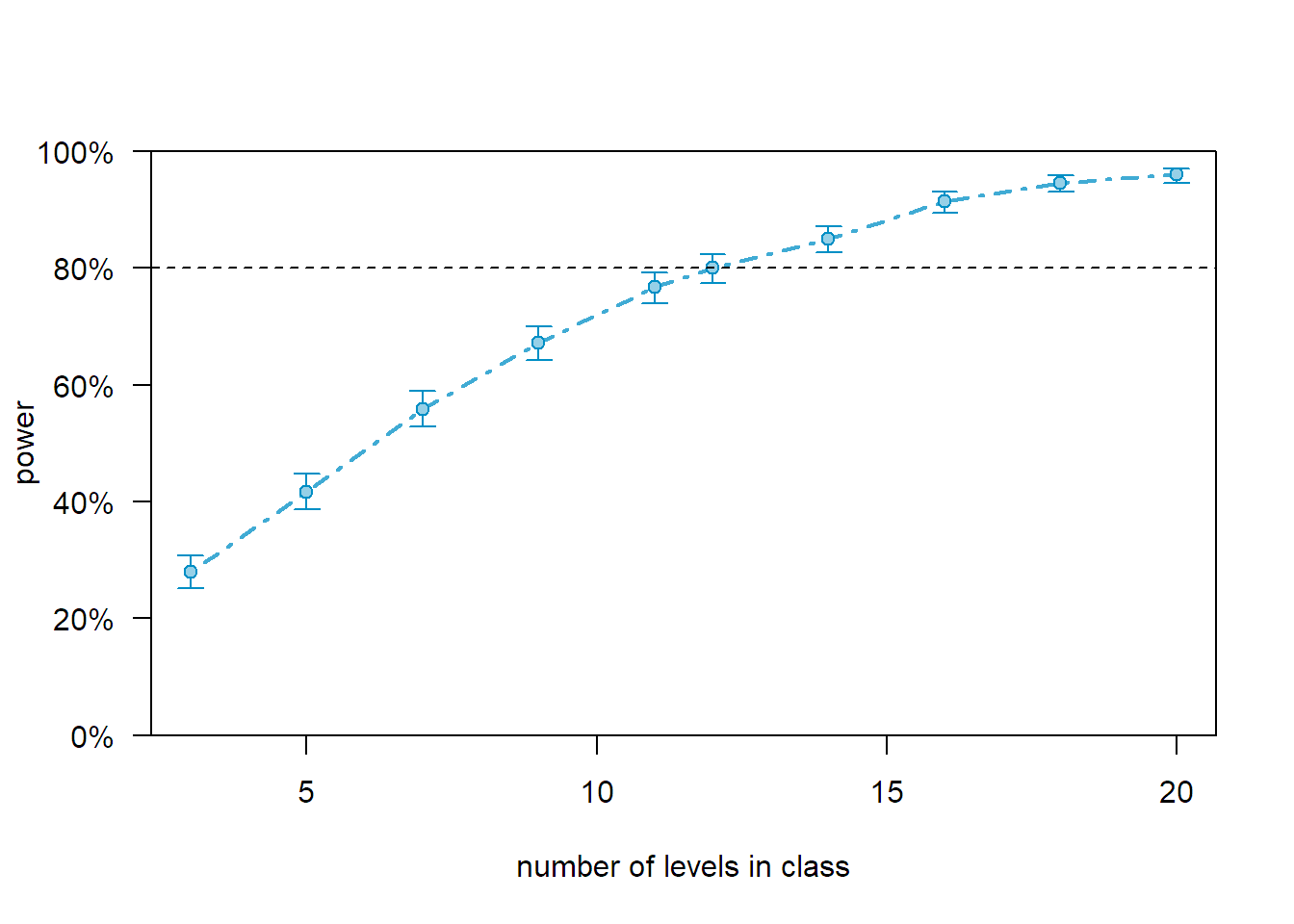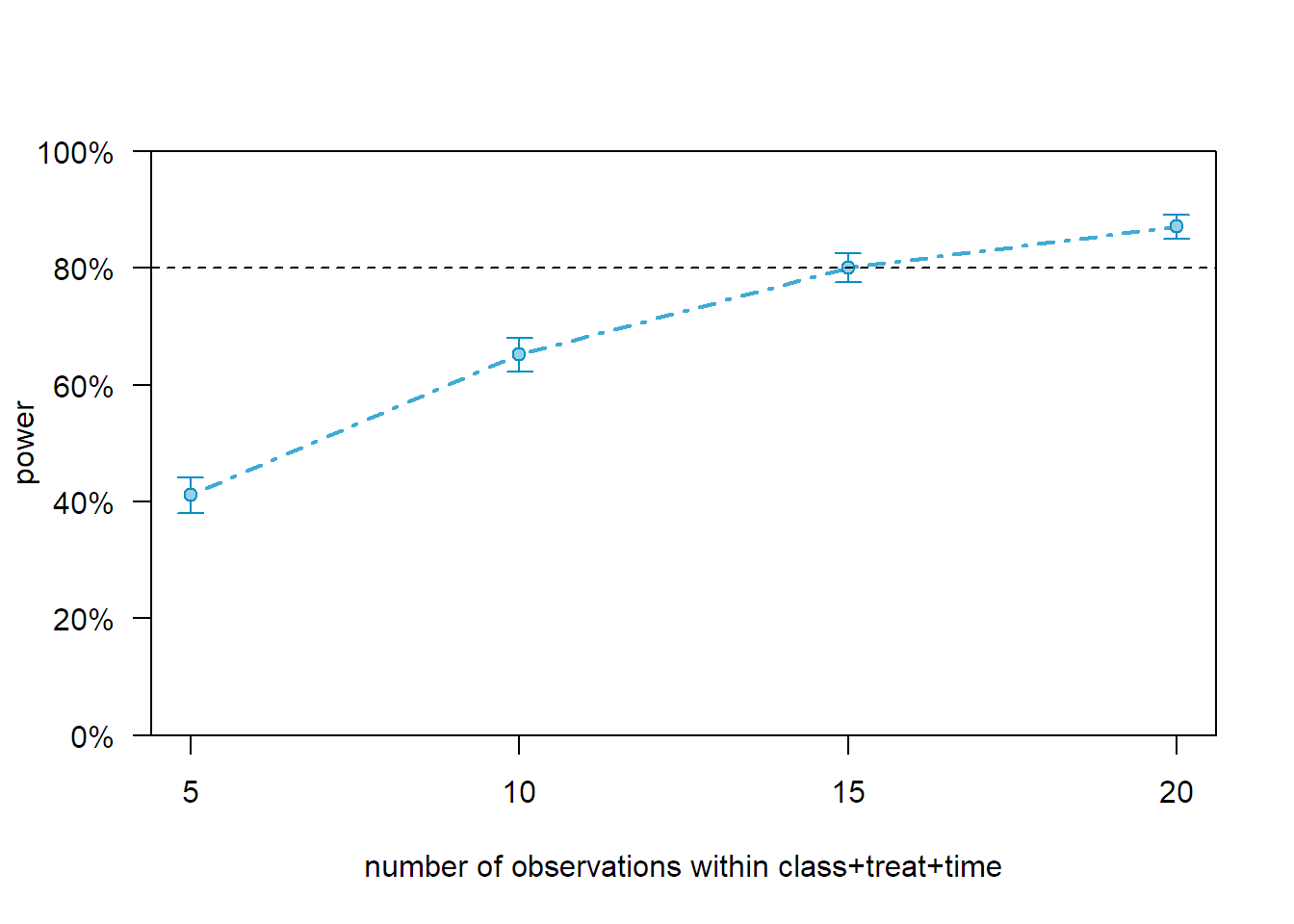# Introduction

library(simr)
## Warning: package 'simr' was built under R version 3.4.4
## Loading required package: lme4
## Warning: package 'lme4' was built under R version 3.4.3
## Loading required package: Matrix
## Warning: package 'Matrix' was built under R version 3.4.3
simrOptions(progress=FALSE)

# Introduction

In this part of the workshop you will use simr to determine power / required sample size for linear mixed effects models. The lme4 package is used for modelling.

# Simulating without data

When pilot data or other closely related data are available it is best to use them to obtain estimates for fixed and random effects. When that is not possible, creating an appropriate model with parameters specified directly still allows the simulation to be carried out. Parameters can be chosen similar to the procedure we discussed for G*Power. Use information from the literature or previous studies as much as possible. As always, you can explore a range of plausible values for important parameters to study the effect they have on the required sample size.

## Create covariates

The first step in creating a model for simulation is to create a set of covariates that the model will be based on.

Example study: Children from different classes at a school will be recruited. Each participant will be allocated to either an intervention or a control group. For each participant measurements will be taken at baseline, immediately following the intervention and at follow-up a few weeks later.

We start by setting up a data frame with 5 classes and 10 participants per class.

subj <- factor(1:10)
class_id <- letters[1:5]
time <- 0:2
group <- c("control", "intervention")

subj_full <- rep(subj, 15)
class_full <- rep(rep(class_id, each=10), 3)
time_full <- rep(time, each=50)
group_full <- rep(rep(group, each=5), 15)

covars <- data.frame(id=subj_full, class=class_full, treat=group_full, time=factor(time_full))

covars

The next step requires us to specify the parameters for the model. We’ll use the model $y \sim \textrm{treatment} + \textrm{time} +\textrm{treatment} \times \textrm{time} + (1|\textrm{class}/\textrm{id}) + \epsilon$

For the purpose of this example parameter values were chosen arbitrarily but should be based on literature or experience as much as possible for real studies.

## Intercept and slopes for intervention, time1, time2, intervention:time1, intervention:time2
fixed <- c(5, 0, 0.1, 0.2, 1, 0.9)

## Random intercepts for participants clustered by class
rand <- list(0.5, 0.1)

## residual variance
res <- 2

## Create model

The makeLmer function from the simr package allows us to combine all this information to create a fitted lmer model from scratch.

model <- makeLmer(y ~ treat*time + (1|class/id), fixef=fixed, VarCorr=rand, sigma=res, data=covars)
model
## Linear mixed model fit by REML ['lmerMod']
## Formula: y ~ treat * time + (1 | class/id)
##    Data: covars
## REML criterion at convergence: 684.4832
## Random effects:
##  Groups   Name        Std.Dev.
##  id:class (Intercept) 0.7071
##  class    (Intercept) 0.3162
##  Residual             2.0000
## Number of obs: 150, groups:  id:class, 50; class, 5
## Fixed Effects:
##             (Intercept)        treatintervention                    time1
##                     5.0                      0.0                      0.1
##                   time2  treatintervention:time1  treatintervention:time2
##                     0.2                      1.0                      0.9

Note that the parameters reported as part of the model summary match the ones we provided, except that we specified random effects as variances and they are reported as standard deviations.

# Power analysis

Once you have a fitted lmer model, whether it was fitted to real data or created from scratch, you can use that to simulate new data and assess the required sample size.

The powerSim function allows us to estimate the power to detect a specific effect in the model. Here we are interested in the effect of the intervention. Since the treatment variable is part of an interaction we will assess its effect by comparing the model specified above to the the alternative model that doesn’t include a treatment variable. We can provide this model alternative via the fcompare function. This allows us to only specify the fixed effects in the alternative model. All random effects will be assumed to be the same as in the original model.

sim_treat <- powerSim(model, nsim=100, test = fcompare(y~time))
sim_treat
## Power for model comparison, (95% confidence interval):
##       41.00% (31.26, 51.29)
##
## Test: Likelihood ratio
##       Comparison to y ~ time + [re]
##
## Based on 100 simulations, (0 warnings, 0 errors)
## alpha = 0.05, nrow = 150
##
## Time elapsed: 0 h 0 m 47 s

We can test for the effect of time in the same way.

sim_time <- powerSim(model, nsim=100, test = fcompare(y~treat))
sim_time
## Power for model comparison, (95% confidence interval):
##       45.00% (35.03, 55.27)
##
## Test: Likelihood ratio
##       Comparison to y ~ treat + [re]
##
## Based on 100 simulations, (0 warnings, 0 errors)
## alpha = 0.05, nrow = 150
##
## Time elapsed: 0 h 0 m 46 s

## Changing the effect size

We can adjust the effect size to suit the analysis, e.g. to compute power for an effect size that is smaller than the one observed in a pilot study, or to study power for a range of effect sizes.

model_large <- model
fixef(model_large)['treatintervention:time1'] <- 2

sim_treat_large <- powerSim(model_large, nsim=100, test = fcompare(y~time))
sim_treat_large
## Power for model comparison, (95% confidence interval):
##       87.00% (78.80, 92.89)
##
## Test: Likelihood ratio
##       Comparison to y ~ time + [re]
##
## Based on 100 simulations, (0 warnings, 0 errors)
## alpha = 0.05, nrow = 150
##
## Time elapsed: 0 h 0 m 42 s

## Changing the number of classes used in study

To study the effect an increase in sample size has on our ability to detect the effect of interest we can increase the number of levels for one of the factors in our model. This can be achieved with the extend function. For example, we could increase the number of classes from 5 to 20.

model_ext_class <- extend(model, along="class", n=20)
model_ext_class
## Linear mixed model fit by REML ['lmerMod']
## Formula: y ~ treat * time + (1 | class/id)
##    Data: covars
## REML criterion at convergence: 684.4832
## Random effects:
##  Groups   Name        Std.Dev.
##  id:class (Intercept) 0.7071
##  class    (Intercept) 0.3162
##  Residual             2.0000
## Number of obs: 150, groups:  id:class, 50; class, 5
## Fixed Effects:
##             (Intercept)        treatintervention                    time1
##                     5.0                      0.0                      0.1
##                   time2  treatintervention:time1  treatintervention:time2
##                     0.2                      1.0                      0.9

We can visualise the effect that varying the number of classes has on the power to detect the intervention effect by asking simr to plot a power curve.

sim_treat_class <- powerSim(model_ext_class, nsim=100, test = fcompare(y~time))
sim_treat_class
## Power for model comparison, (95% confidence interval):
##       98.00% (92.96, 99.76)
##
## Test: Likelihood ratio
##       Comparison to y ~ time + [re]
##
## Based on 100 simulations, (0 warnings, 0 errors)
## alpha = 0.05, nrow = 600
##
## Time elapsed: 0 h 0 m 59 s
p_curve_treat <- powerCurve(model_ext_class, test=fcompare(y~time), along="class")
plot(p_curve_treat)## Changing the number of participants per class

Instead of increasing the number of classes we could increase the number of participants per class. This can be achieved with the within argument to extend. Here we are extending the number of students for each treatment at each time point within each class to 20.

model_ext_subj <- extend(model, within="class+treat+time", n=20)
model_ext_subj
## Linear mixed model fit by REML ['lmerMod']
## Formula: y ~ treat * time + (1 | class/id)
##    Data: covars
## REML criterion at convergence: 684.4832
## Random effects:
##  Groups   Name        Std.Dev.
##  id:class (Intercept) 0.7071
##  class    (Intercept) 0.3162
##  Residual             2.0000
## Number of obs: 150, groups:  id:class, 50; class, 5
## Fixed Effects:
##             (Intercept)        treatintervention                    time1
##                     5.0                      0.0                      0.1
##                   time2  treatintervention:time1  treatintervention:time2
##                     0.2                      1.0                      0.9

Once again we can obtain a power estimate at the increased sample size as well as a power curve that combines estimates at different sample sizes.

sim_treat_subj <- powerSim(model_ext_subj, nsim=100, test = fcompare(y~time))
sim_treat_subj
## Power for model comparison, (95% confidence interval):
##       88.00% (79.98, 93.64)
##
## Test: Likelihood ratio
##       Comparison to y ~ time + [re]
##
## Based on 100 simulations, (0 warnings, 0 errors)
## alpha = 0.05, nrow = 600
##
## Time elapsed: 0 h 0 m 42 s
p_curve_treat <- powerCurve(model_ext_subj, test=fcompare(y~time), within="class+treat+time", breaks=c(5,10,15,20))
plot(p_curve_treat)## Changing number of classes and participants per class

We may want to study models with changes to multiple sample size components. The relevant changes can be applied to the model successively.

model_final <- extend(model, along="class", n=8)
model_final <- extend(model_final, within="class+treat+time", n=10)

sim_final <- powerSim(model_final, nsim=100, test = fcompare(y~time))
sim_final
## Power for model comparison, (95% confidence interval):
##       91.00% (83.60, 95.80)
##
## Test: Likelihood ratio
##       Comparison to y ~ time + [re]
##
## Based on 100 simulations, (0 warnings, 0 errors)
## alpha = 0.05, nrow = 480
##
## Time elapsed: 0 h 1 m 19 s